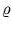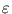Next: 3.4.2 Results Up: 3.4 Digit classification Previous: 3.4 Digit classification

## 3.4.1 Methods

The digits were taken from the MNIST database (LeCun, 1998), which is a subset of the NIST database produced by the U.S. National Institute of Standards and Technology (appendix D). The database contains 60 000 images of digits for training and 10 000 for testing. The gray-scale images (scaled to pixel values in the interval [0, 1]) are centered in a 28×28 pixel grid.

Two training sets were generated (Möller and Hoffmann, 2004). One contained the original 28×28 images transformed into 784 dimensional vectors. The other one was composed of subsampled images of size 8×8 transformed into 64 dimensional vectors. The subsampled images were obtained by removing a margin of 4 pixels, such that the digits in the resulting 20×20 image fitted tightly into the frame. Each of the pixels of the final 8×8 image was produced by a weighted summation over a local region, using a Gaussian weight function with a half-width of 1.25 pixels (in the 20×20 image). This second training set used only the first 1000 images of each digit3.2. Each training set was split into ten parts, one part for each digit.

Each digit was trained by one model, separately. The local PCA mixture models contained ten units with ten principal components. Both NGPCA and NGPCA-constV used the parameter set: tmax = 30 000,(0) = 2,(tmax) = 0.002,(0) = 0.5,(tmax) = 0.0002.

The results for the 28×28 training set were compared to a single PCA, which extracted 40 principal components for each digit. The single PCA had fewer parameters then the mixture model because higher numbers of principal components did not improve the classification (using more components makes the ellipsoids thicker, thus ellipsoids from different digits probably overlapp). Moreover, the results were compared to standard Neural Gas, which contained 109 code-book vectors (as many as required to obtain about the same number of free parameters as for the local PCA mixture). Neural Gas used the same training parameters as NGPCA.

To classify a digit, the error measure (3.2) was computed for all units of the ten fitted models, and the digit was assigned to the model that comprised the unit with the minimal error value. In the standard Neural Gas case, the Euclidean distance was used instead of (3.2).Next: 3.4.2 Results Up: 3.4 Digit classification Previous: 3.4 Digit classification
Heiko Hoffmann
2005-03-22